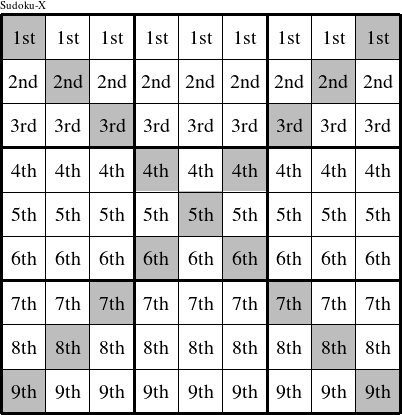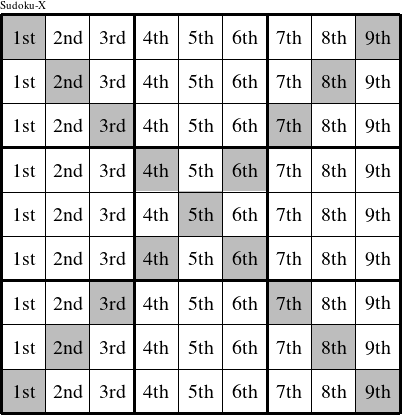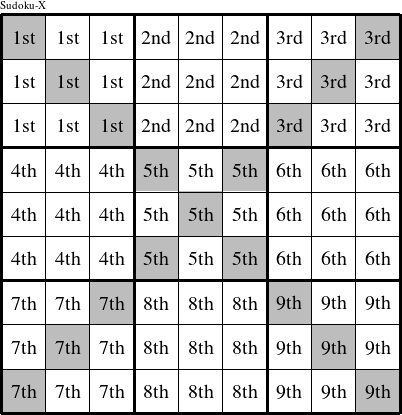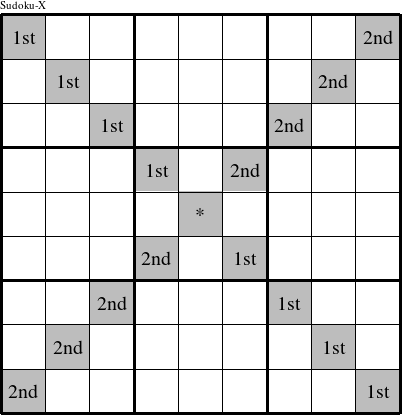#### Printable version

Detailed instructions for working a group puzzle of type 'Education-X'

This is a signature puzzle named 'Education-X'

The 9 elements in each group of this type of puzzle are: E, D, U, C, A, T, I, O, and N.

Every group in the puzzle must contain exactly this set of elements.

####In a Education-X puzzle, each row is a group numbered as shown in the figure above.

####In a Education-X puzzle, each column is a group numbered as shown in the figure above.

####In a Education-X puzzle, each 3x3 square is a group numbered as shown in the figure above.

####In a Education-X puzzle, each diagonal is a group numbered as shown in the figure above.